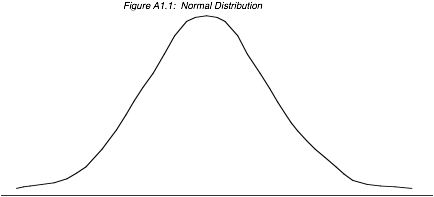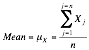# Summarizing Data

Large amounts of data are often compressed into more easily assimilated summaries, which provide the user with a sense of the content, without overwhelming him or her with too many numbers. There a number of ways data can be presented. We will consider two here—one is to present the data in a distribution, and the other is to provide summary statistics that capture key aspects of the data.

## Data Distributions

When presented with thousands of pieces of information, you can break the numbers down into individual values (or ranges of values) and indicate the number of individual data items that take on each value or range of values. This is called a frequency distribution. If the data can only take on specific values, as is the case when we record the number of goals scored in a soccer game, you get a discrete distribution. When the data can take on any value within the range, as is the case with income or market capitalization, it is called a continuous distribution.

The advantages of presenting the data in a distribution are twofold. For one thing, you can summarize even the largest data sets into one distribution and get a measure of what values occur most frequently and the range of high and low values. The second is that the distribution can resemble one of the many common ones about which we know a great deal in statistics. Consider, for instance, the distribution that we tend to draw on the most in analysis: the normal distribution, illustrated in Figure A1.1.A normal distribution is symmetric, has a peak centered around the middle of the distribution, and tails that are not fat and stretch to include infinite positive or negative values.  Not all distributions are symmetric, though. Some are weighted towards extreme positive values and are called positively skewed, and some towards extreme negative values and are considered negatively skewed. Figure A1.2 illustrates positively and negatively skewed distributions.

## Summary Statistics

The simplest way to measure the key characteristics of a data set is to estimate the summary statistics for the data. For a data series, X1, X2, X3, . . . Xn, where n is the number of observations in the series, the most widely used summary statistics are as follows:

The mean (m), which is the average of all of the observations in the data series.The median, which is the midpoint of the series; half the data in the series is higher than the median and half is lower.

The variance, which is a measure of the spread in the distribution around the mean and is calculated by first summing up the squared deviations from the mean, and then dividing by either the number of observations (if the data represent the entire population) or by this number, reduced by one (if the data represent a sample).The standard deviation is the square root of the variance.

The mean and the standard deviation are the called the first two moments of any data distribution. A normal distribution can be entirely described by just these two moments; in other words, the mean and the standard deviation of a normal distribution suffice to characterize it completely. If a distribution is not symmetric, the skewness is the third moment that describes both the direction and the magnitude of the asymmetry and the kurtosis (the fourth moment)  measures the fatness of the tails of the distribution relative to a normal distribution.